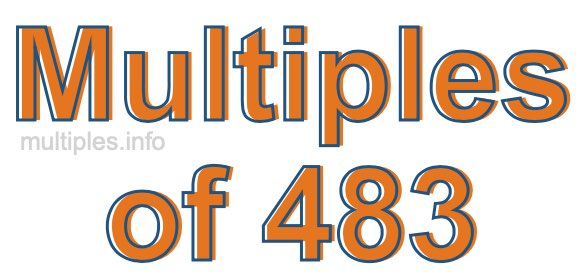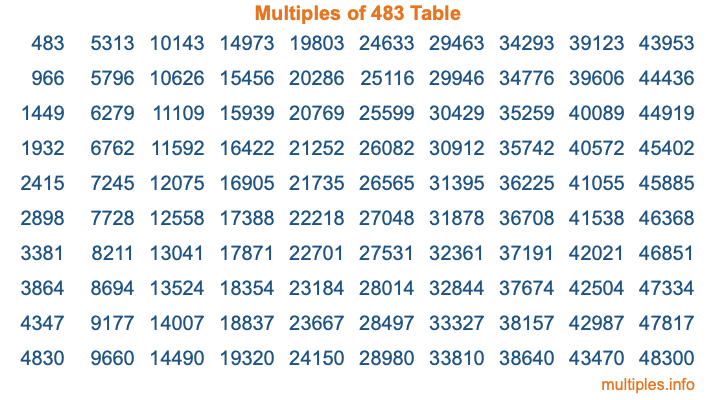Multiples of 483Welcome to the Multiples of 483 page. Here we will first teach you everything you will ever need to know about the multiples of 483, and then give you a study guide summary of everything we taught you to make sure you remember it all. Use this page to look up facts and learn information about the multiples of 483. This page will make you a multiples of four hundred eighty-three expert!

Definition of Multiples of 483
Multiples of 483 are all the numbers that when divided by 483 equal an integer. Each of the multiples of 483 are called a multiple. A multiple of 483 is created by multiplying 483 by an integer.

Therefore, to create a list of multiples of 483, you start with 1 multiplied by 483, then 2 multiplied by 483, then 3 multiplied by 483, and so on for as long as you want. Thus, the list of the first five multiples of 483 is 483, 966, 1449, 1932, and 2415. To see a larger list of multiples of 483, see the printable image of Multiples of 483 further down on this page. We also have a category where you can choose any nth multiple of 483.

Multiples of 483 Checker
The Multiples of 483 Checker below checks to see if any number of your choice is a multiple of 483. In other words, it checks to see if there is any number (integer) that when multiplied by 483 will equal your number. To do that, we divide your number by 483. If the the quotient is an integer, then your number is a multiple of 483.

Is  a multiple of 483?

Least Common Multiple of 483 and ...
A Least Common Multiple (LCM) is the lowest multiple that two or more numbers have in common. This is also called the smallest common multiple or lowest common multiple and is useful to know when you are adding our subtracting fractions. Enter one or more numbers below (483 is already entered) to find the LCM.

Check out our LCM Calculator if you need more details about the Least Common Multiple or if you need the LCM for different numbers for adding and subtraction fractions.

nth Multiple of 483
As we stated above, 483 is the first multiple of 483, 966 is the second multiple of 483, 1449 is the third multiple of 483, and so on. Enter a number below to find the nth multiple of 483.

th multiple of 483

Multiples of 483 vs Factors of 483
483 is a multiple of 483 and a factor of 483, but that is where the similarities end. All postive multiples of 483 are 483 or greater than 483. All positive factors of 483 are 483 or less than 483.

Below is the beginning list of multiples of 483 and the factors of 483 so you can compare:

Multiples of 483: 483, 966, 1449, 1932, 2415, etc.

Factors of 483: 1, 3, 7, 21, 23, 69, 161, 483

As you can see, the multiples of 483 are all the numbers that you can divide by 483 to get a whole number. The factors of 483, on the other hand, are all the whole numbers that you can multiply by another whole number to get 483.

It's also interesting to note that if a number (x) is a factor of 483, then 483 will also be a multiple of that number (x).

Multiples of 483 vs Divisors of 483
The divisors of 483 are all the integers that 483 can be divided by evenly. Below is a list of the divisors of 483.

Divisors of 483: 1, 3, 7, 21, 23, 69, 161, 483

The interesting thing to note here is that if you take any multiple of 483 and divide it by a divisor of 483, you will see that the quotient is an integer.

Multiples of 483 Table
Below is an image of the first 100 multiples of 483 in a table. The table is in chronological order, column by column. The first column has the first ten multiples of 483, the second column has the next ten multiples of 483, and so on.The Multiples of 483 Table is also referred to as the 483 Times Table or Times Table of 483. You are welcome to print out our table for your studies.

Negative Multiples of 483
Although not often discussed or needed in math, it is worth mentioning that you can make a list of negative multiples of 483 by multiplying 483 by -1, then by -2, then by -3, and so on, to get the following list of negative multiples of 483:

-483, -966, -1449, -1932, -2415, etc.

Multiples of 483 Summary
Below is a summary of important Multiples of 483 facts that we have discussed on this page. To retain the knowledge on this page, we recommend that you read through the summary and explain to yourself or a study partner why they hold true.

There are an infinite number of multiples of 483.

A multiple of 483 divided by 483 will equal a whole number.

483 divided by a factor of 483 equals a divisor of 483.

The nth multiple of 483 is n times 483.

The largest factor of 483 is equal to the first positive multiple of 483.

483 is a multiple of every factor of 483.

483 is a multiple of 483.

A multiple of 483 divided by a divisor of 483 equals an integer.

483 divided by a divisor of 483 equals a factor of 483.

Any integer times 483 will equal a multiple of 483.

Multiples of a Number
Here you can get the multiples of another number, all with the same attention to detail as we did for multiples of 483 on this page.

Multiples of
Multiples of 484
Did you find our page about multiples of four hundred eighty-three educational? Do you want more knowledge? Check out the multiples of the next number on our list!